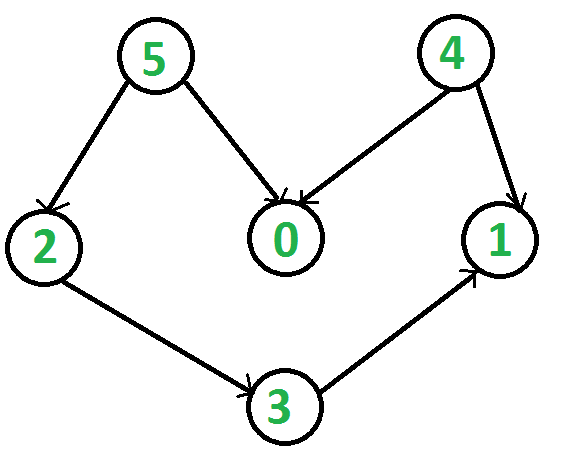# Python Program for Topological Sorting

Topological sorting for Directed Acyclic Graph (DAG) is a linear ordering of vertices such that for every directed edge uv, vertex u comes before v in the ordering. Topological Sorting for a graph is not possible if the graph is not a DAG.

For example, a topological sorting of the following graph is “5 4 2 3 1 0”. There can be more than one topological sorting for a graph. For example, another topological sorting of the following graph is “4 5 2 3 1 0”. The first vertex in topological sorting is always a vertex with in-degree as 0 (a vertex with no in-coming edges).`#Python program to print topological sorting of a DAG ` `from` `collections ``import` `defaultdict ` ` `  `#Class to represent a graph ` `class` `Graph: ` `    ``def` `__init__(``self``,vertices): ` `        ``self``.graph ``=` `defaultdict(``list``) ``#dictionary containing adjacency List ` `        ``self``.V ``=` `vertices ``#No. of vertices ` ` `  `    ``# function to add an edge to graph ` `    ``def` `addEdge(``self``,u,v): ` `        ``self``.graph[u].append(v) ` ` `  `    ``# A recursive function used by topologicalSort ` `    ``def` `topologicalSortUtil(``self``,v,visited,stack): ` ` `  `        ``# Mark the current node as visited. ` `        ``visited[v] ``=` `True` ` `  `        ``# Recur for all the vertices adjacent to this vertex ` `        ``for` `i ``in` `self``.graph[v]: ` `            ``if` `visited[i] ``=``=` `False``: ` `                ``self``.topologicalSortUtil(i,visited,stack) ` ` `  `        ``# Push current vertex to stack which stores result ` `        ``stack.insert(``0``,v) ` ` `  `    ``# The function to do Topological Sort. It uses recursive  ` `    ``# topologicalSortUtil() ` `    ``def` `topologicalSort(``self``): ` `        ``# Mark all the vertices as not visited ` `        ``visited ``=` `[``False``]``*``self``.V ` `        ``stack ``=``[] ` ` `  `        ``# Call the recursive helper function to store Topological ` `        ``# Sort starting from all vertices one by one ` `        ``for` `i ``in` `range``(``self``.V): ` `            ``if` `visited[i] ``=``=` `False``: ` `                ``self``.topologicalSortUtil(i,visited,stack) ` ` `  `        ``# Print contents of stack ` `        ``print` `stack ` ` `  `g``=` `Graph(``6``) ` `g.addEdge(``5``, ``2``); ` `g.addEdge(``5``, ``0``); ` `g.addEdge(``4``, ``0``); ` `g.addEdge(``4``, ``1``); ` `g.addEdge(``2``, ``3``); ` `g.addEdge(``3``, ``1``); ` ` `  `print` `"Following is a Topological Sort of the given graph"` `g.topologicalSort() ` `#This code is contributed by Neelam Yadav `

Output:

```Following is a Topological Sort of the given graph
5 4 2 3 1 0```

Please refer complete article on Topological Sorting for more details!

My Personal Notes arrow_drop_up
Article Tags :

1

Please write to us at contribute@geeksforgeeks.org to report any issue with the above content.